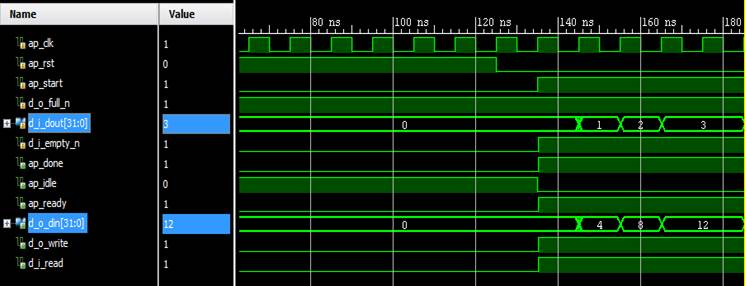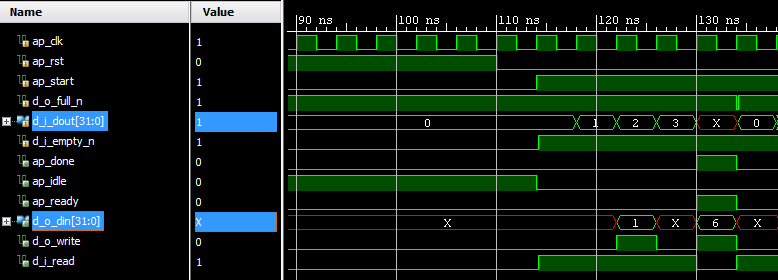# AR# 60434

## Description

This Answer Record describes the "volatile" data type with an example for Vivado HLS.

The behavior is the same as the "C" behavior.

## Solution

Volatile Type:

The volatile qualifier impacts how many reads or writes are performed in the RTL when pointers are accessed multiple times on function interfaces.

Limitation:

Arbitrary precision types do not support the volatile qualifier for arithmetic operations. Any arbitrary precision data types using the volatile qualifier must be assigned to a non-volatile data type before being used in arithmetic expression

Recommendation:

You must use the volatile qualifier on any function argument accessed multiple times.

The volatile qualifier tells the C compiler (and Vivado HLS) to make no assumptions about the pointer accesses. That is, the data is volatile and may change.

Example:

Target is to achieve 4 reads and 2 writes as a multi-pointer access. Data types are C integers of typedef "int".

Directives used in both case:

#pragma HLS INTERFACE ap_fifo depth=4 port=d_i

#pragma HLS INTERFACE ap_fifo depth=2 port=d_o

 Without Volatile: void pointer_stream_bad ( dout_t *d_o, din_t *d_i) { din_t acc = 0; acc += *d_i; acc += *d_i; *d_o = acc; acc += *d_i; acc += *d_i; *d_o = acc; }   Cosim result: Din         Dout 0              0 1              4 2              8 3              12 Waveform:With Volatile: void pointer_stream_better ( volatile dout_t *d_o, volatile din_t *d_i) { din_t acc = 0; acc += *d_i; acc += *d_i; *d_o = acc; acc += *d_i; acc += *d_i; *d_o = acc; }   Cosim Result: Din         Dout 0               1 1               6 2 3   Waveform:Vivado HLS example for volatile type:

Open Example Project> Coding Style Examples> pointer_stream_better

With reference to the 2014.1 version, please refer to page 368: